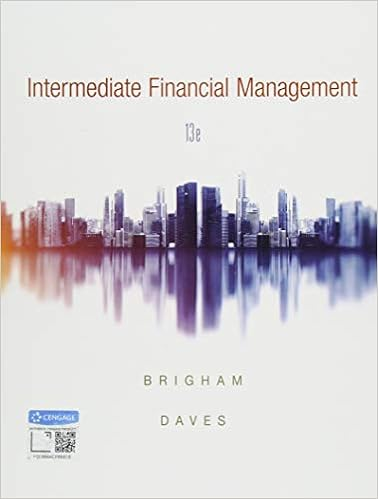# Session 23 - Accounting 102 Session 23 Variance Analysis...

• Test Prep
• 31

This preview shows page 1 - 8 out of 31 pages.

##### We have textbook solutions for you!
The document you are viewing contains questions related to this textbook.The document you are viewing contains questions related to this textbook.
Chapter 12 / Exercise 12-5
Intermediate Financial Management
Brigham/DavesExpert Verified
Accounting 102 Session 23
##### We have textbook solutions for you!
The document you are viewing contains questions related to this textbook.The document you are viewing contains questions related to this textbook.
Chapter 12 / Exercise 12-5
Intermediate Financial Management
Brigham/DavesExpert Verified
Variance Analysis – Costs
Objectives of Cost Variance Analysis Provide a better understanding of the reasons why actual costs differed from expected costs, and assigning appropriate responsibility for these differences What might cause a manager to spend more than budgeted? Who is responsible for higher-than-expected raw material prices? Who is responsible for excess scrap (leading to higher material usage) in manufacturing? Cost Variance = [Actual Costs - Expected Costs] A cost variance is Favorable if Actual < Expected A cost variance is Unfavorable if Actual > Expected
Required Cost Information The development of a static or master budget at the beginning of a period requires the following information: Expected production output (EO) in number of units Expected or standard inputs (SI) per unit (e.g., direct labor hours per unit, pounds of material per unit) Expected or standard prices (SP) for each resource (e.g., hourly labor rate, price per pound of materials) Expected cost per unit of output = SI x SP Computation of actual costs at the end of the period requires the following information: • Actual production output (AO) in number of units • Actual inputs (AI) per unit • Actual prices (AP) for each resource Actual cost per unit of output = AI x AP
Steps to Analyze Direct Cost Variances Static Budget Direct Cost Plan Step 1 Change Output From Planned To Actual Step 2 Change Input Quantity From Planned To Actual Step 3 Change Input Price From Planned To Actual Output Volume Adjusted Cost Plan Input Quantity Adjusted Cost Plan Input Price Adjusted Cost Plan Output Quantity Plan (EO) Actual (AO) Actual (AO) Actual (AO) Input Quantity Plan (SI) Plan (SI) Actual (AI) Actual (AI) Input Price/Cost Plan (SP) Plan (SP) Plan (SP) Actual (AP) Sales Revenue Planned Cost Output Volume Adjusted Cost Input Quantity Adjusted Cost Input Price Adjusted Cost Volume Variance Efficiency Variance Price Variance Flexible Budget Variance Static Budget Variance
6 Breakdown of Cost Variances Static Budget Variance Volume Variance Flexible Budget Variance Price Variance Efficiency Variance
Analysis of Cost Variances, An Example
•••